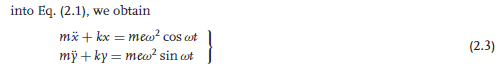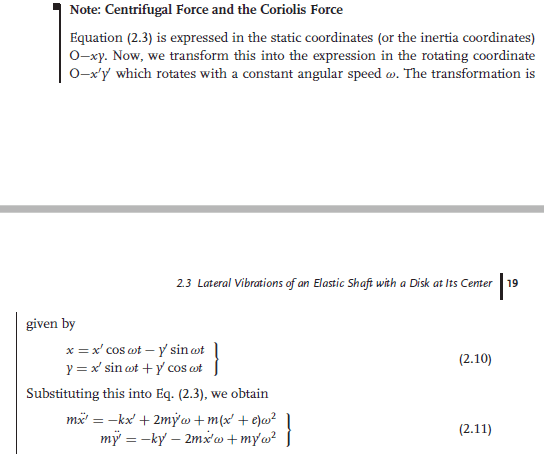# Natural frequency in stationary and rotating frames....

• tricha122
In summary, the damping force is not transformed in the equations of motion, which is why the amplitude vs. input frequency chart shows the same results for the rotating and stationary frames.f

#### tricha122

Hi,

I am trying to gain insight into using stationary vs. rotating coordinate frames for natural frequency calculations. I have seen many FE codes suggest that critical frequencies can be calculated differently in rotating and inertial frames, so i wanted to do a 1D calc to see for myself how things could be different.

I am trying to model a 1D spring-mass-damper with an rotating unbalance input force in both frames, and solve them numerically for a sweep of input frequencies to view the output displacement vs. frequency. And i expect to see a sharp increase where w = sqrt(K/M)

The equations of motion i am solving are as follows:

Stationary Frame:

M*x'' + C*x' + K*x = m*e*w^2*cos(w*t)
M*y'' + C*y' + K*y = m*e*w^2*sin(w*t)

these equations can be solved independently

For the rotating frame, i apply the transformation:
x = x1*cos(w*t) - y1*sin(w*t)
y = x1*sin(w*t) + y1*cos(w*t)

where x1, y1 are in the rotating frame, x1 is along the radius, y1 is "circumferential"

when i plug in this transformation, and simplify i get the following equations:

Mx1'' = -Kx1 - C(x1'-y1*w) + 2*M*y1'*w+m*(x1+e)*w^2
My1'' = -Ky1 - C(y1'+x1*w) - 2*M*x1'*w+m*y1*w^2

where the equations cannot be solved independently due to the coriolis force. (CF force shows up too).

I solved both stationary & rotating frame equations using M = 200, K = 104000, C = 10, m = 1, w = variable over a long "time" so that the damping could get rid of the "particular solution". After a long "time" i output the max amplitude. This resulted in an amplitude vs. input frequency chart

The stationary frame showed a sharp increase at w = ~22 = (sqrt(104000/200)) as expected.
The rotating frame showed no sharp increase, just steady rising with the forcing function. The strange part however was that at low frequencies, the results were identical to the stationary frame.

Can anyone shed some light on this? i have attached an image of my output. I also uploaded text files of the MATLAB code i was running.

#### Attachments

• Frame_of_Reference_Result.xlsx
12.6 KB · Views: 222
• SMDRF.txt
1.2 KB · Views: 525
• SMDRFsweep.txt
348 bytes · Views: 514
• SMDSF.txt
1 KB · Views: 478
• SMDSFsweep.txt
348 bytes · Views: 514
when i plug in this transformation, and simplify i get the following equations
Just checking: can you show your work, or at least state that you also describe the driving forces in the rotating frame ?

Just checking: can you show your work, or at least state that you also describe the driving forces in the rotating frame ?

The driving forces are transformed as a result of the equations of motion being transformed.

The reference below [Linear and Nonlinear Rotordynamic by Ishida & Yamamoto] shows the equations of motion - they are the same as mine with the exception that i added damping terms to the inertial frame prior to transforming.#### Attachments

Ok, so driving force is transformed. How about damping force ?